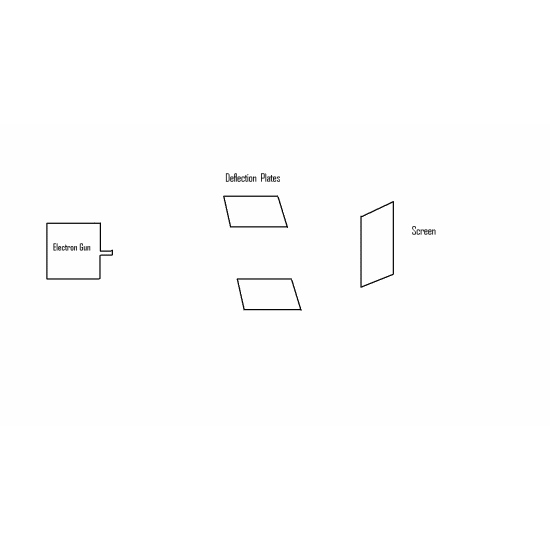# Charge

2009-06-01

Charge is a characteristic unit of matter.

In an atom an electric charge occurs whenever the number of protons in nucleus is greater than the number of electrons surrounding that nucleus. If the number of protons is greater than the number of electrons then the atom is said to be positively charged. If the number of electrons is greater than the number of protons then the atom is said to be negatively charged.

Now just think are you using a charge right now.? the answer is yes.

The monitor right in front of you uses a charge to emit light of various colours.It also controls the light so that it appears in the form of words and symbols in front of you.(If you are using a CRT monitor.. Not in the case of LCD monitor).

Now lets see interesting animated representation of charge.

We know that a charge can be classified as

• Negative charge-Electron
• Positive charge-Proton
• Neutral or no charge-Neutron

In the below representation an electron is released or triggered from electron gun.

• Consider a CRT monitor. Here if we want to display a dot in centre of screen the deflection plates are not given any charge.The electron travels a flat path.
• Now if the dot has to appear in top of screen (or) above the centre positive charge is given to the top plate.So the electron is attracted by positive charge in top plate and repelled by negative charge in bottom plate.
• If the dot has to appear below the centre(or) bottom of screen,. positive charge is given to bottom plate and negative charge is given to top plate. Pictorial Representation is shown below.Now let us define Coulombs Inverse square law for charges:

When two charges are separated by a distance r between them,then the force is directly proportional to the product magnitude of charges and inversely proportional to square of distance between them.

Let q1,q2 be two charges.separated by a distance r .

F= q1q2/(4*pi*e*r^2) Where e( epsilon) is the permittivity.